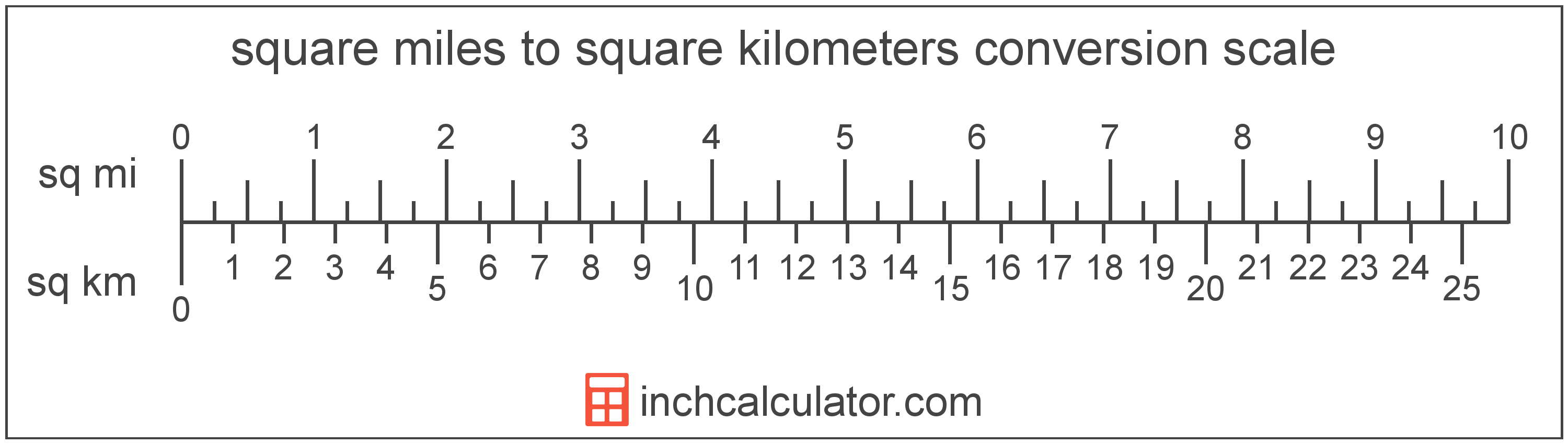# Square Miles to Square Kilometers Conversion

Enter the area in square miles below to get the value converted to square kilometers.

Results in Square Kilometers:1 sq mi = 2.589988 sq km

## How to Convert Square Miles to Square KilometersTo convert a square mile measurement to a square kilometer measurement, multiply the area by the conversion ratio.

Since one square mile is equal to 2.589988 square kilometers, you can use this simple formula to convert:

square kilometers = square miles × 2.589988

The area in square kilometers is equal to the square miles multiplied by 2.589988.

For example, here's how to convert 5 square miles to square kilometers using the formula above.
5 sq mi = (5 × 2.589988) = 12.949941 sq km

## Square Miles

One square mile is equal to the area of a square with sides that are 1 mile long. One square mile is roughly equal to 2.59 square kilometers.

The square mile is a US customary and imperial unit of area. Square miles can be abbreviated as sq mi, and are also sometimes abbreviated as mi². For example, 1 square mile can be written as 1 sq mi or 1 mi².

## Square Kilometers

One square kilometer is equal to the area of a square with sides that are 1 kilometer in length.

The square kilometer, or square kilometre, is a multiple of the square meter, which is the SI derived unit for area. In the metric system, "kilo" is the prefix for 103. A square kilometer is sometimes also referred to as a square km. Square kilometers can be abbreviated as sq km, and are also sometimes abbreviated as km². For example, 1 square kilometer can be written as 1 sq km or 1 km².

## Square Mile to Square Kilometer Conversion Table

Square mile measurements converted to square kilometers
Square Miles Square Kilometers
1 sq mi 2.59 sq km
2 sq mi 5.18 sq km
3 sq mi 7.77 sq km
4 sq mi 10.36 sq km
5 sq mi 12.95 sq km
6 sq mi 15.54 sq km
7 sq mi 18.13 sq km
8 sq mi 20.72 sq km
9 sq mi 23.31 sq km
10 sq mi 25.9 sq km
11 sq mi 28.49 sq km
12 sq mi 31.08 sq km
13 sq mi 33.67 sq km
14 sq mi 36.26 sq km
15 sq mi 38.85 sq km
16 sq mi 41.44 sq km
17 sq mi 44.03 sq km
18 sq mi 46.62 sq km
19 sq mi 49.21 sq km
20 sq mi 51.8 sq km
21 sq mi 54.39 sq km
22 sq mi 56.98 sq km
23 sq mi 59.57 sq km
24 sq mi 62.16 sq km
25 sq mi 64.75 sq km
26 sq mi 67.34 sq km
27 sq mi 69.93 sq km
28 sq mi 72.52 sq km
29 sq mi 75.11 sq km
30 sq mi 77.7 sq km
31 sq mi 80.29 sq km
32 sq mi 82.88 sq km
33 sq mi 85.47 sq km
34 sq mi 88.06 sq km
35 sq mi 90.65 sq km
36 sq mi 93.24 sq km
37 sq mi 95.83 sq km
38 sq mi 98.42 sq km
39 sq mi 101.01 sq km
40 sq mi 103.6 sq km